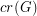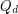# Guy, Richard K.

## The Crossing Number of the Hypercube ★★

Author(s): Erdos; Guy

The crossing numberofis the minimum number of crossings in all drawings ofin the plane.

The-dimensional (hyper)cubeis the graph whose vertices are all binary sequences of length, and two of the sequences are adjacent inif they differ in precisely one coordinate.

ConjectureKeywords: crossing number; hypercube